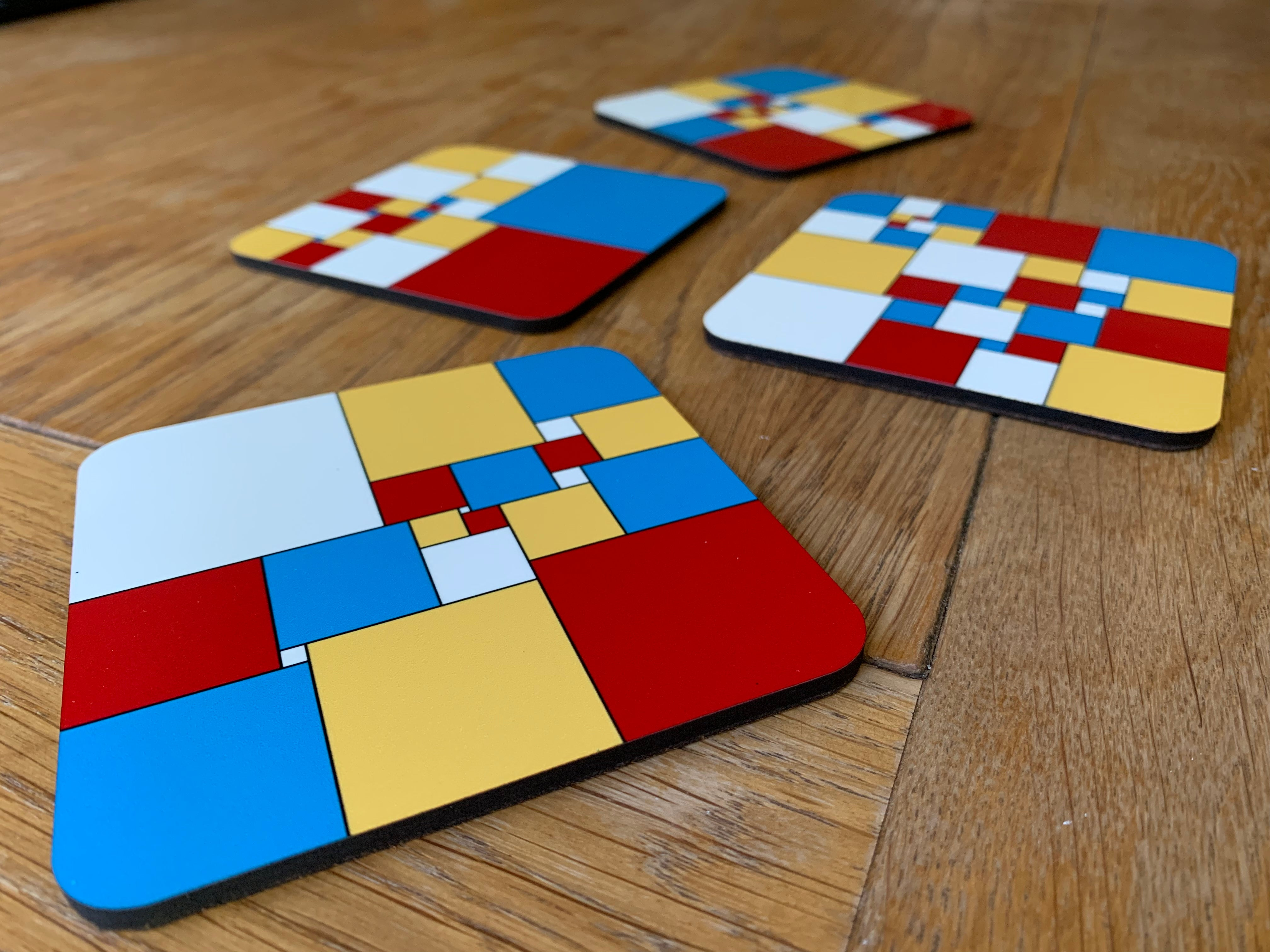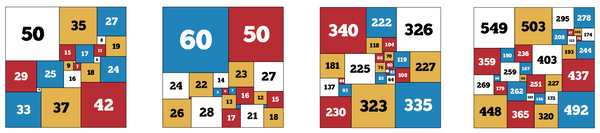1# Squared Square Coasters

## \$21.00

You may have heard of squaring the circle - but what about squaring the square?

A square made from smaller squares is known as a squared square. The hard bit is that the sides of each square need to be an integer and the squares have to be all different sizes!

These coasters are some of our favourites, and have been selected by mathematician James Grime.The set includes (L-R) a lowest order squared square (made from the fewest squares), smallest size squared square, a square square with a centre square exactly in the middle, and the nicest squared square (smallest ratio between the largest and smallest square).

The four coasters can be combined to make another valid squared square, or used to protect surfaces from hot and cold drinks.

Set of four high quality hardwood backed cork coasters, with a booklet explaining the maths and showing the sizes of each square.

Watch James talk about squared squares in this video:

Price = p251 pence = £15.97